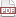### Bayes v Kolmogorov

23 October 14. [link]PDF version

$\def\Re{{\mathbb R}} \def\datas{{\mathbb D}} \def\params{{\mathbb P}} \def\models{{\mathbb M}} \def\mod#1{M_{#1}}$

We have a likelihood function that takes two inputs, which we will name the data and the parameter, and which gives the nonnegative likelihood of that combination, $L: d, p \to \Re^+$. [I wrote a lot of apropos things about this function in an early blog post, by the way.]

The two inputs are symmetric in the sense that we could slice the function either way. Fixing $p=\rho$ defines a one-parameter function $L_\rho: d\to \Re^+$; fixing $d=\delta$ defines a one-parameter function $L_\delta: p \to \Re^+$.

But the inputs are not symmetric in a key way, which I will call the unitary axiom (it doesn't seem to have a standard name). It's one of Kolmogorov's axioms for constructing probability measures. The axiom states that, given a fixed parameter, some value of $d$ will be observed with probability one. That is, \begin{equation} \int_{\forall \delta} L_\rho(\delta) d\delta = 1, \forall \rho. \end{equation} In plain language, when we live in a world where there is one fixed underlying parameter, one data point or another will be observed with probability one.

This is a strong statement, because we read the total density as an indication of the likelihood of the parameter taking on the given value. I tell you that $p=3$, and we check the likelihood and see that the total density on that state of the world is one. Then you tell me that, no, $p=4$, and we refer to $L(d, 4)$, and see that it integrates to one as well.

Somebody else comes along and points out that this may work for discrete-valued $p$, but a one-dimensional slice isn't the right way to read a continuous density, insisting that we consider only ranges of parameters, such as $p\in[2.75,3.25]$ or $p \in [3.75,4.25]$. But if the integral over a single slice is always one, then the double integral is easy: $\int_{\rho\in[2.75,3.25]}\int_{\forall \delta} L(\delta, \rho) d\delta d\rho$ $=\int_{\rho\in[2.75,3.25]} 1 d\rho$ $=.5$, and the same holds for $p \in [3.75,4.25]$. We're in the same bind, unable to use the likelihood function to put more density on one set of parameters compared to any other of the same size.

This rule is asymmetric, by the way, because if we had all the parameters in the universe, whatever that might mean, and a fixed data set $\delta$, then $\int_{\forall \rho} L_\delta(\rho) d\rho$ could be anything.

Of course, we don't have all the data in the universe. Instead, we gather a finite quantity of data, and find the more likely parameter given that subset of the data. For example, we might observe the data set $\Delta=\{2, 3, 4\}$ and use that to say something about a parameter $\mu$. I don't want to get into specific functional forms, but for the sake of discussion, say that $L(\Delta, 2)=.1$; $L(\Delta, 3)=.15$; $L(\Delta, 4)=.1$. We conclude that three is the most likely value of $\mu$.

What if we lived in an alternate universe where the unitary axiom didn't hold? Given a likelihood function $L(d, p)$ that conforms to the unitary axiom, let $$L'(d, p)\equiv L(d, p)\cdot f(p),$$ where $f(p)$ is nonnegative and finite but otherwise anything. Then the total density on $\rho$ given all the data in the universe is $\int_{\forall \delta} L_{\rho}(\delta)f(\rho) d\delta = f(\rho)$.

For the sake of discussion, let $f(2)=.1$, $f(3)=.2$, $f(4)=.4$. Now, when we observe $\Delta=\{2, 3, 4\}$, $L'(\Delta, 2)=.01$, $L'(\Delta, 3)=.03$, $L'(\Delta, 4)=.04$, and we conclude that $\mu=4$ is the most likely value of $p$.

Bayesian updating is typically characterized as a composition of two functions, customarily named the prior and the likelihood. In the notation here, these are $f(p)$ and $L(d, p)$. Without updating, all values of $p$ are equally likely in the world described by $L$, until data is gathered. The prior breaks the unitary axiom, and specifies that, even without gathering data, some values of $p$ are more likely than others. When we do gather data, our prior belief that some values of $p$ are more likely than others advises our beliefs.

Our belief about the relative preferability of one value of $p$ over another could be summarized into a proper distribution, but once again, there is no unitary axiom requiring that a distribution over the full parameter space integrate to one. For example, the bridge from the Bayesian-updated story to the just-a-likelihood story is the function $f(\rho)=1, \forall \rho$. This is an improper distribution, but it does express that each value of $p$ has the same relative weight.

In orthodox practice, everything we write down about the data follows the unitary axiom. For a given observation, $L'(\delta, p)$ is a function of one variable, sidestepping any issues about integrating over the space of $d$. We may require that this univariate function integrate to one, or just stop after stating that $L'(\delta, p) \propto f(p)L(\delta, p)$, because we usually only care about ratios of the form $L'(\delta, \rho_1)/L'(\delta, \rho_2)$, in which case rescaling is a waste of time.

In a world where all parameters are observable and fixed, the unitary axiom makes so much sense it's hard to imagine not having it. But in a meta-world where the parameter has different values in different worlds, the unitary axiom implies that all worlds have an equal slice of the likelihood's density. We usually don't believe this implication, and Bayesian updating is our way of side-stepping it.